# Python Program to Merge Two Lists

• Merge two lists of 6-6 elements
• Merge two lists of given size of elements
• Merge two lists in ascending order
• Merge two lists in descending order

## Merge Two Lists of Equal Elements

The question is, write a Python program that merges two lists entered by user. Here is its answer. This program receives 6-6 elements for both lists and then merges the lists into third one. Let's have a look at the program and its sample run:

```listOne = []
listTwo = []

print("Enter 6 Elements for First List: ")
for i in range(6):
listOne.append(input())
print("\nEnter 6 Elements for Second List: ")
for i in range(6):
listTwo.append(input())

listThree = []
for i in range(6):
listThree.append(listOne[i])
for i in range(6):
listThree.append(listTwo[i])

print("\nThe New (Merged) List is: ")
print(listThree)```

Here is the initial output produced by this program: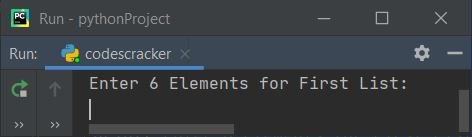Now supply inputs say 1, 2, 3, 4, 5, 6 as six elements for first and 7, 8, 9, 10, 11, 12 as six elements for second list, to merge these two lists and print the new merged list as given below: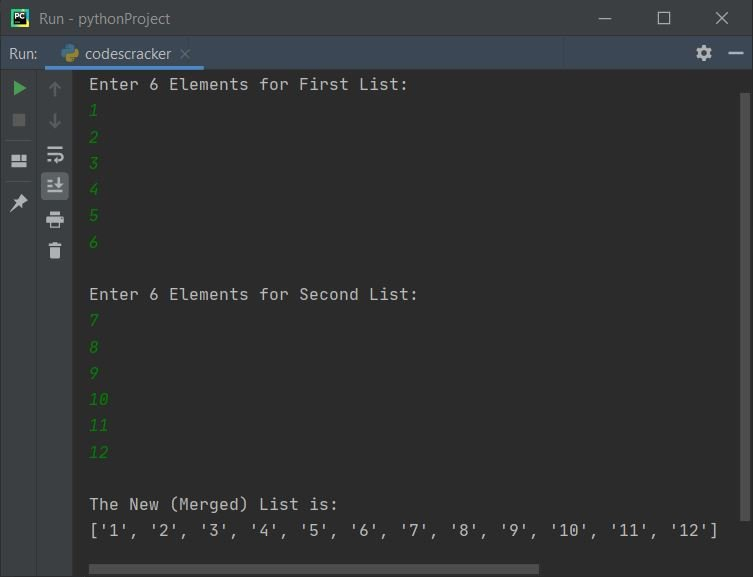## Merge Two Lists of Given Size of Elements

This program is similar to previous one but with some extra features such as, user is allowed to define the size of both the list before entering elements for it. Also, end= is used to skip insertion of an automatic newline using print(), and str() converts any value to a string type.

```one = []
two = []

print(end="Enter the Size of First List: ")
oneSize = int(input())
print(end="Enter " +str(oneSize)+ " Elements: ")
for i in range(oneSize):
one.append(input())

print(end="\nEnter the Size of Second List: ")
twoSize = int(input())
print(end="Enter " +str(twoSize)+ " Elements: ")
for i in range(twoSize):
two.append(input())

three = one+two
threeSize = len(three)

print("\nThe Two Lists Merged Successfully!")
print("\nThe New List is:-")
for i in range(threeSize):
print(end=three[i] + " ")
print()```

Here is its sample run with user input, 5 as size of first list, then c, o, d, e, s as five elements of first list, now 7 as size, then c, r, a, c, k, e, r as seven elements of second lists: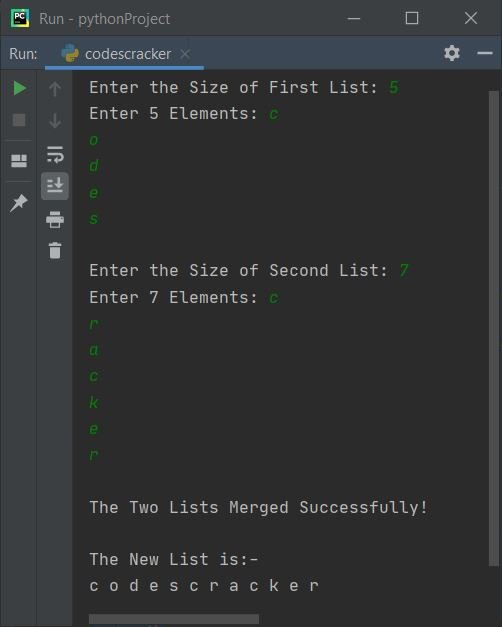## Merge Two Lists and Sort it

To merge two lists and sort it before printing the final merged list on output, just use sort() method in this way:

`three.sort()`

before printing the elements of list, three.

## Merge Two List in Ascending Order

This program merges two lists and then sorts the list in ascending order before printing the elements of merged list using sort() method as told above:

```one = []
two = []

print(end="Enter First List's Size: ")
oneSize = int(input())
print(end="Enter " +str(oneSize)+ " Elements: ")
for i in range(oneSize):
one.append(input())

print(end="\nEnter Second List's Size: ")
twoSize = int(input())
print(end="Enter " +str(twoSize)+ " Elements: ")
for i in range(twoSize):
two.append(input())

three = one+two
threeSize = len(three)
three.sort()

print("\nThe New List is:-")
for i in range(threeSize):
print(end=str(three[i]) + " ")
print()```

Here is its sample run with user input, 6 as size and 10, 9, 8, 7, 1, 6 as six elements of first list, 4 as size and 5, 4, 3, 2 as four elements of second list: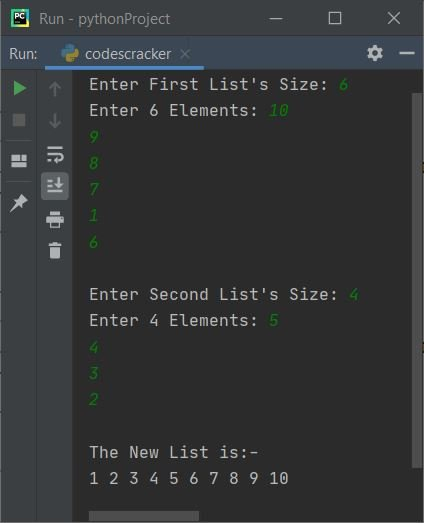### Merge Two Lists in Descending Order

To merge two lists in descending order, just reverse the list after sorting it using reverse() method. Here is the complete version of the program:

```listOne = list()
listTwo = list()

print(end="Enter First List's Size: ")
listOneSize = int(input())
print(end="Enter " + str(listOneSize) + " Elements: ")
for i in range(listOneSize):
listOne.append(input())

print(end="\nEnter Second List's Size: ")
listTwoSize = int(input())
print(end="Enter " + str(listTwoSize) + " Elements: ")
for i in range(listTwoSize):
listTwo.append(input())

listThree = listOne + listTwo
listThreeSize = len(listThree)
listThree.sort()
listThree.reverse()

print("\nThe New List is:-")
for i in range(listThreeSize):
print(end=str(listThree[i]) + " ")
print()```

Here is its sample run with user input, 3 as size and 5, 1, 4 as elements of first list, 3 as size and 3, 0, 2 as elements of second list: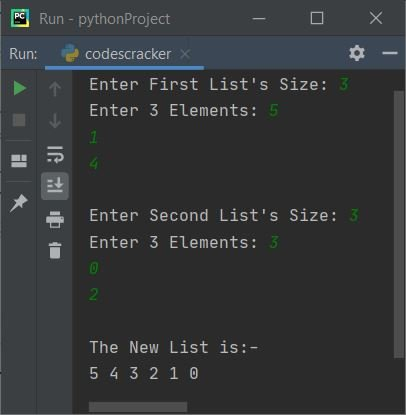Python Online Test

« Previous Program Next Program »